# Gradient Boosting Regression Analysis Reveals Dependent Variables and Interrelationships

15 de Mar de 2021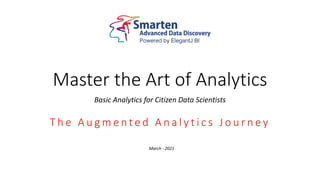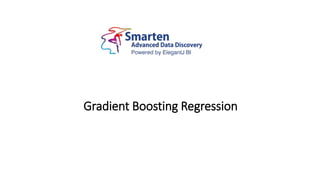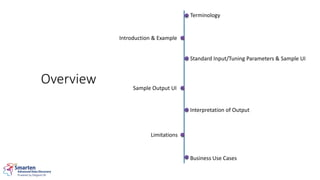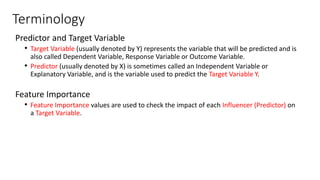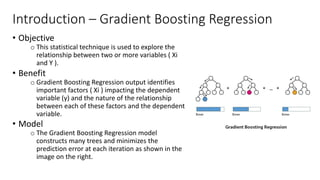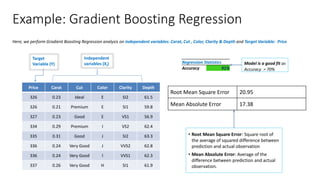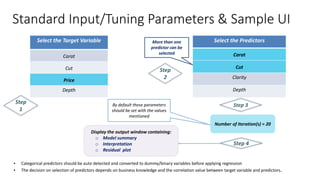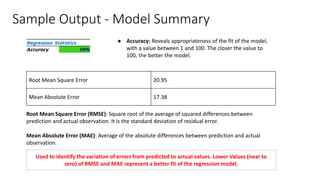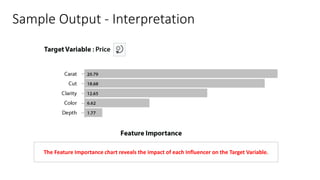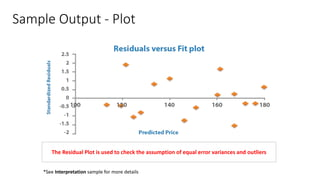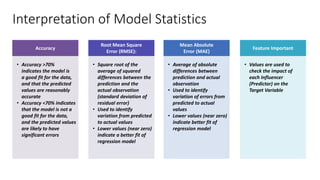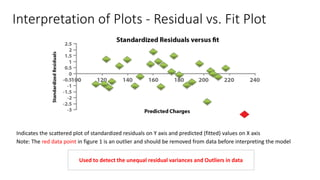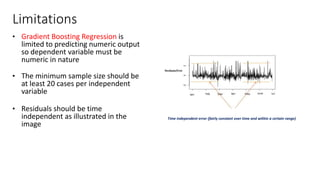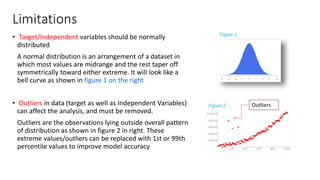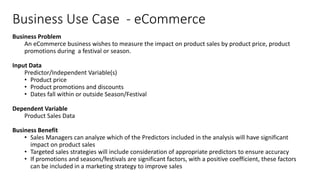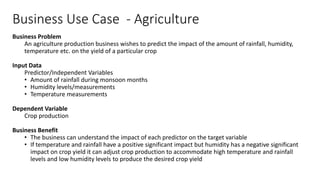1 de 17

### Gradient Boosting Regression Analysis Reveals Dependent Variables and Interrelationships

• 1. Master the Art of Analytics Basic Analytics for Citizen Data Scientists T h e A u g m e n t e d A n a l y t i c s J o u r n e y March - 2021
• 3. Terminology Introduction & Example Standard Input/Tuning Parameters & Sample UI Sample Output UI Interpretation of Output Limitations Business Use Cases Overview
• 4. Terminology Predictor and Target Variable • Target Variable (usually denoted by Y) represents the variable that will be predicted and is also called Dependent Variable, Response Variable or Outcome Variable. • Predictor (usually denoted by X) is sometimes called an Independent Variable or Explanatory Variable, and is the variable used to predict the Target Variable Y. Feature Importance • Feature Importance values are used to check the impact of each Influencer (Predictor) on a Target Variable.
• 5. Introduction – Gradient Boosting Regression • Objective o This statistical technique is used to explore the relationship between two or more variables ( Xi and Y ). • Benefit o Gradient Boosting Regression output identifies important factors ( Xi ) impacting the dependent variable (y) and the nature of the relationship between each of these factors and the dependent variable. • Model o The Gradient Boosting Regression model constructs many trees and minimizes the prediction error at each iteration as shown in the image on the right.
• 6. Example: Gradient Boosting Regression Price Carat Cut Color Clarity Depth 326 0.23 Ideal E SI2 61.5 326 0.21 Premium E SI1 59.8 327 0.23 Good E VS1 56.9 334 0.29 Premium I VS2 62.4 335 0.31 Good J SI2 63.3 336 0.24 Very Good J VVS2 62.8 336 0.24 Very Good I VVS1 62.3 337 0.26 Very Good H SI1 61.9 Target Variable (Y) Independent variables (Xi) Model is a good fit as Accuracy > 70% Regression Statistics Accuracy 91% • Root Mean Square Error: Square root of the average of squared difference between prediction and actual observation • Mean Absolute Error: Average of the difference between prediction and actual observation. Root Mean Square Error 20.95 Mean Absolute Error 17.38 Here, we perform Gradient Boosting Regression analysis on independent variables: Carat, Cut , Color, Clarity & Depth and Target Variable: Price
• 7. Select the Target Variable Carat Cut Price Depth Step 1 Select the Predictors Carat Cut Clarity Depth Step 2 More than one predictor can be selected Step 3 Number of Iteration(s) = 20 By default these parameters should be set with the values mentioned Step 4 Display the output window containing: o Model summary o Interpretation o Residual plot ▪ Categorical predictors should be auto detected and converted to dummy/binary variables before applying regression ▪ The decision on selection of predictors depends on business knowledge and the correlation value between target variable and predictors. Standard Input/Tuning Parameters & Sample UI
• 8. Sample Output - Model Summary ● Accuracy: Reveals appropriateness of the fit of the model, with a value between 1 and 100. The closer the value to 100, the better the model. Root Mean Square Error (RMSE): Square root of the average of squared differences between prediction and actual observation. It is the standard deviation of residual error. Mean Absolute Error (MAE): Average of the absolute differences between prediction and actual observation. Root Mean Square Error 20.95 Mean Absolute Error 17.38 Used to identify the variation of errors from predicted to actual values. Lower Values (near to zero) of RMSE and MAE represent a better fit of the regression model.
• 9. Sample Output - Interpretation The Feature Importance chart reveals the impact of each Influencer on the Target Variable.
• 10. Sample Output - Plot *See Interpretation sample for more details The Residual Plot is used to check the assumption of equal error variances and outliers
• 11. Interpretation of Model Statistics Accuracy • Accuracy >70% indicates the model is a good fit for the data, and that the predicted values are reasonably accurate • Accuracy <70% indicates that the model is not a good fit for the data, and the predicted values are likely to have significant errors Root Mean Square Error (RMSE): • Square root of the average of squared differences between the prediction and the actual observation (standard deviation of residual error) • Used to identify variation from predicted to actual values • Lower values (near zero) indicate a better fit of regression model Mean Absolute Error (MAE) • Average of absolute differences between prediction and actual observation • Used to identify variation of errors from predicted to actual values • Lower values (near zero) indicate better fit of regression model Feature Important • Values are used to check the impact of each Influencer (Predictor) on the Target Variable
• 12. Interpretation of Plots - Residual vs. Fit Plot Indicates the scattered plot of standardized residuals on Y axis and predicted (fitted) values on X axis Note: The red data point in figure 1 is an outlier and should be removed from data before interpreting the model Used to detect the unequal residual variances and Outliers in data
• 13. Limitations • Gradient Boosting Regression is limited to predicting numeric output so dependent variable must be numeric in nature • The minimum sample size should be at least 20 cases per independent variable • Residuals should be time independent as illustrated in the image Time independent error (fairly constant over time and within a certain range)
• 14. Limitations • Target/Independent variables should be normally distributed A normal distribution is an arrangement of a dataset in which most values are midrange and the rest taper off symmetrically toward either extreme. It will look like a bell curve as shown in figure 1 on the right • Outliers in data (target as well as Independent Variables) can affect the analysis, and must be removed. Outliers are the observations lying outside overall pattern of distribution as shown in figure 2 in right. These extreme values/outliers can be replaced with 1st or 99th percentile values to improve model accuracy Outliers Figure 1 Figure 2
• 15. Business Use Case - eCommerce Business Problem An eCommerce business wishes to measure the impact on product sales by product price, product promotions during a festival or season. Input Data Predictor/Independent Variable(s) • Product price • Product promotions and discounts • Dates fall within or outside Season/Festival Dependent Variable Product Sales Data Business Benefit • Sales Managers can analyze which of the Predictors included in the analysis will have significant impact on product sales • Targeted sales strategies will include consideration of appropriate predictors to ensure accuracy • If promotions and seasons/festivals are significant factors, with a positive coefficient, these factors can be included in a marketing strategy to improve sales
• 16. Business Use Case - Agriculture Business Problem An agriculture production business wishes to predict the impact of the amount of rainfall, humidity, temperature etc. on the yield of a particular crop Input Data Predictor/Independent Variables • Amount of rainfall during monsoon months • Humidity levels/measurements • Temperature measurements Dependent Variable Crop production Business Benefit • The business can understand the impact of each predictor on the target variable • If temperature and rainfall have a positive significant impact but humidity has a negative significant impact on crop yield it can adjust crop production to accommodate high temperature and rainfall levels and low humidity levels to produce the desired crop yield